• 因此为了保证数据满足上述统计方法的应用条件，对原始数据进行正态性检验是十分必要的，这一节内容我们主要向大家介绍如何对数据资料进行正态性检验。 一、正态性检验：偏度和峰度 1、偏度（Skewness）：描述...
当我们应用统计方法对数据进行分析时，会发现许多计量资料的分析方法，例如常用的T检验、方差分析、相关分析以及线性回归等等，都要求数据服从正态分布或者近似正态分布，但这一前提条件往往被使用者所忽略。因此为了保证数据满足上述统计方法的应用条件，对原始数据进行正态性检验是十分必要的，这一节内容我们主要向大家介绍如何对数据资料进行正态性检验。

一、正态性检验：偏度和峰度

1、偏度（Skewness）：描述数据分布不对称的方向及其程度（见图1）。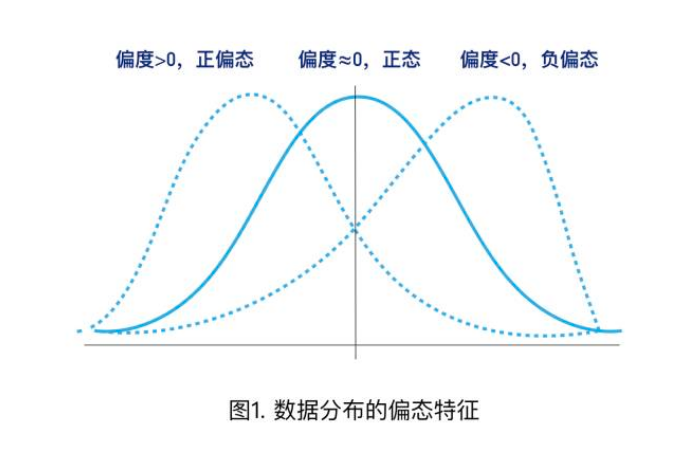当偏度≈0时，可认为分布是对称的，服从正态分布；

当偏度>0时，分布为右偏，即拖尾在右边，峰尖在左边，也称为正偏态；

当偏度<0时，分布为左偏，即拖尾在左边，峰尖在右边，也称为负偏态；

注意：数据分布的左偏或右偏，指的是数值拖尾的方向，而不是峰的位置，容易引起误解。

2、峰度（Kurtosis）：描述数据分布形态的陡缓程度（图2）。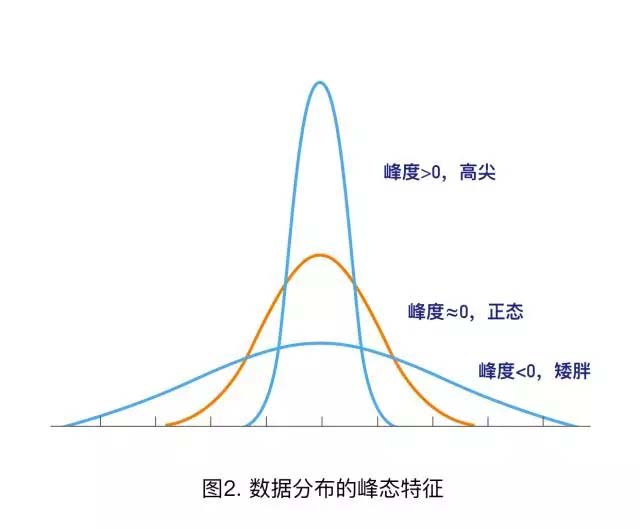当峰度≈0时，可认为分布的峰态合适，服从正态分布（不胖不瘦）；

当峰度>0时，分布的峰态陡峭（高尖）；

当峰度<0时，分布的峰态平缓（矮胖）；

利用偏度和峰度进行正态性检验时，可以同时计算其相应的Z评分（Z-score），即：偏度Z-score=偏度值/标准误，峰度Z-score=峰度值/标准误。在α=0.05的检验水平下，若Z-score在±1.96之间，则可认为资料服从正态分布。

了解偏度和峰度这两个统计量的含义很重要，在对数据进行正态转换时，需要将其作为参考，选择合适的转换方法。

3、SPSS操作方法

以分析某人群BMI的分布特征为例。

文章剩余内容<<<<


展开全文SPSS教程
• 笔者之前写了题为《用Python讲解偏度和峰度》的文章，在那篇文章里，笔者介绍了偏度、峰度以及如何基于二者进行数据正态性判断，而今天笔者将介绍一下如何将数据进行正态性转换。在我们进行数...笔者之前写了题为《用Python讲解偏度和峰度》的文章，在那篇文章里，笔者介绍了偏度、峰度以及如何基于二者进行数据正态性的判断，而今天笔者将介绍一下如何将数据进行正态性转换。在我们进行数据分析时，遇到的数据往往不是呈正态分布的，而如果数据不是正态性的，那么在部分情况下会带来一些问题。比如某些模型的前提就是要求数据具有正态性（KNN、贝叶斯等），此外数据具有正态性可以在一定程度上提高机器学习的训练效果。那么将非正态分布的数据转为正态分布有哪些方法呢？笔者在这里简单介绍几种常见的方法。第一种是对数据取平方根，这种方法常用于泊松分布数据，第二种是取对数，在对数正态分布数据中常用，这两种方法都要求数据非负，当然可以给这些数据都加一个常数使其为正值。还有一种方法是对数据取到数，常用于有极值的情况。但笔者今天介绍的方法和上面三种都不同，这种方法名为BOX-COX变换法。之所以介绍这种方法，是因为很多读者对这种方法不是太熟悉，想让大家更好地了解一下，同时这种方法在金融领域应用较多，笔者之前做过一些金融方面的数据分析，所以把这种方法拿出来重点介绍一下。下面首先还是对BOX-COX变换做一个简单的介绍。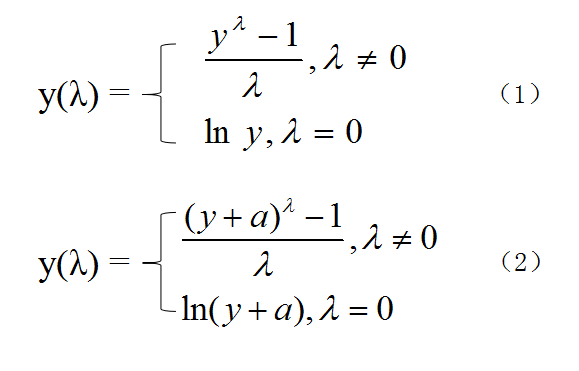图1. BOX-COX变换的公式BOX-COX变换是由博克斯（Box）与考克斯（Cox）在1964年提出的一种非常广泛的变换方法，它是对因变量y作如图1中式（1）的变换，式中λ是待定参数。此变换要求y的各分量都大于0，否则就要用另一种方式作BOX-COX变换，如图1中式（2）所示。这种方法先对y做平移，使y+a的各个分量都大于0，然后再做BOX-COX变换。对于不同的λ，所作的变换也不同，所以这是一个变换族，它包含一些常用操作，如对数变换（λ=0），平方根变换（λ=0.5），以及倒数变换（λ=-1）。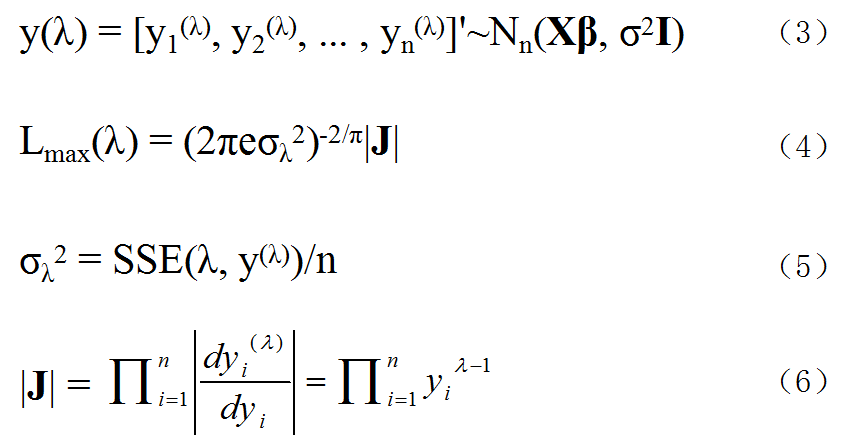图2. BOX-COX变换的部分推导公式通过此变换，我们寻找合适的λ，使得变换后图2中式（3）符合线性回归模型的各项假设：误差各分量等方差、不相关等。事实上，BOX-COX变换不仅可以处理异方差问题，还能处理自相关、误差非正态、回归函数非线性等情况。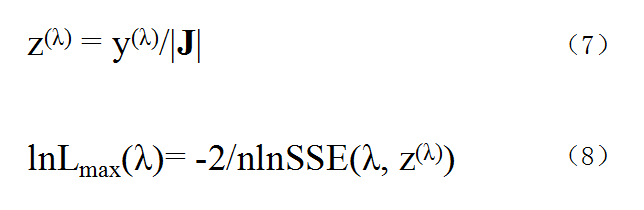图3. BOX-COX变换的部分推导公式经过计算可得λ的最大似然估计为图2中式（4），而式（5）和（6）也都与（4）相关联。而通过图3中式（7）的变换，我们可以得到式（8）。为找出λ，使得Lmax(λ)最大，只需使SSE最小即可，它的解析解比较难找，通常是给出一系列λ的值，计算相应的SSE，取最小SSE所对应的λ即可。在了解了BOX-COX变换基本原理之后我们就直接用python代码来说明一下其具体应用。在scipy的stats模块当中直接集成了BOX-COX方法，所以我们就利用该方法。首先还是导入各种库。import numpy as np
import pandas as pd
import seaborn as sns
from scipy import stats
import matplotlib.pyplot as plt
笔者在这里将用两个例子来说明BOX-COX变换的应用。首先我们来看一个比较特殊的例子。这个例子来自帕累托分布，帕累托分布原理如图4所示，其在实际生活中非常常见，比如石油的储量、社会财富的分布、人类居住区的大小等都是近似帕累托分布。我们首先生成服从帕累托分布的数据，然后再作出分布直方图。这里stats.lomax(c=14).rvs(size=500)这行代码中，lomax方法就是scipy中生成帕累托数据的方法，c=14是生成某个状态（或者说是形状）的数据，这个值可以随意设置。rvs是生成数据的方法，这里生成500个数值。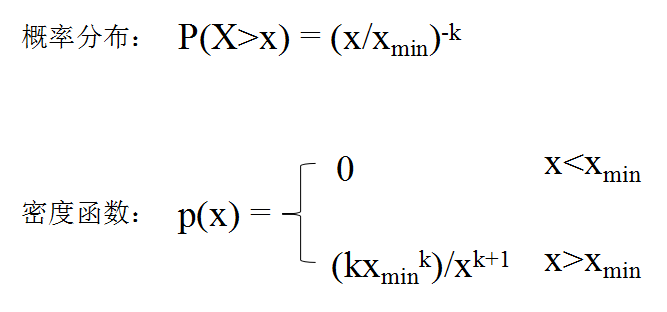图4. 帕累托分布介绍fig, ax = plt.subplots(figsize=[12,8])
data1 = stats.lomax(c=14).rvs(size=500) #生成帕累托分布数据，数据每次生成时可能不同
sns.distplot(data1)
plt.show()
结果如图5所示。从图中我们可以明显看出，该数据的分布不服从正态分布，而是一个右偏分布，数据比较集中于0到2之间。接下来我们对其进行BOX-COX变换，代码如下。这里stats.boxcox就是对原数据进行转换，会返回两个值，第一个值就是转换后的数据，所以我们取第一个值。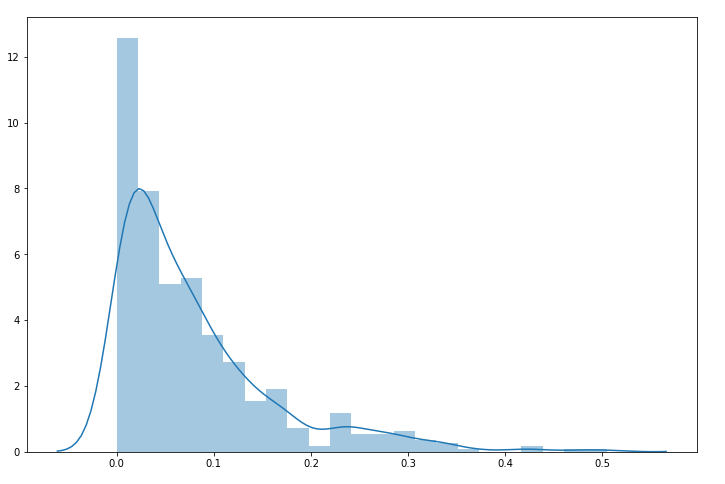图5. 帕累托数据直方图fig, ax = plt.subplots(figsize=[12,8])
converted_data1 = stats.boxcox(data1) #对数据进行BOX-COX变换
sns.distplot(converted_data1)
plt.show()
结果如图6所示。从图中可以看出，该分布已经有了很明显的正态分布的特征，接下来我们再进一步验证一下。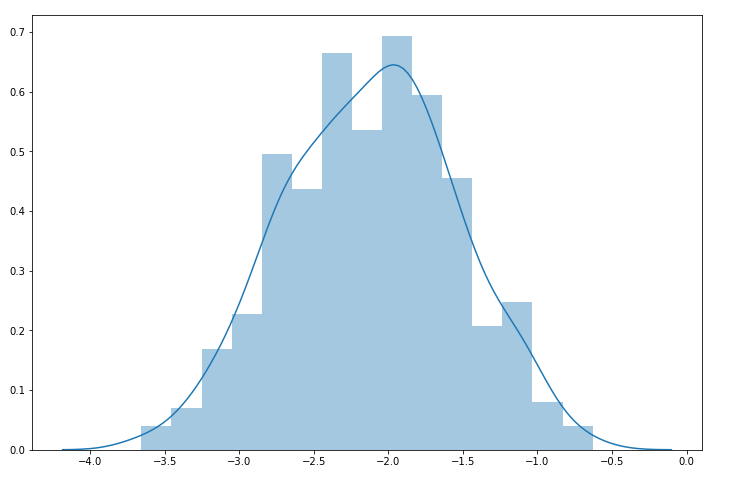图6. 转换后的帕累托数据直方图fig, ax = plt.subplots(figsize=[12,8])
prob = stats.probplot(converted_data1, dist=stats.norm, plot=ax) #生成Q-Q图
plt.show()
结果如图7所示。图7是一个Quantile-Quantile Plot，就是我们平常所说的Q-Q图。Q-Q图是将一组数据作为参考，另一组数据作为样本，样本数据每个值在样本数据集中的百分位数（percentile）作为其在Q-Q图上的横坐标值，而该值放到参考数据集中时的百分位数作为其在Q-Q图上的纵坐标。一般我们会在Q-Q图上做一条45度的参考线。如果两组数据来自同一分布，那么样本数据集的点应该都落在参考线附近；反之如果距离越远，这说明这两组数据很可能来自不同的分布。可以看到绝大部分数据就分布于这条红线附近，可以判断我们转换过后的数据已经是服从正态分布了。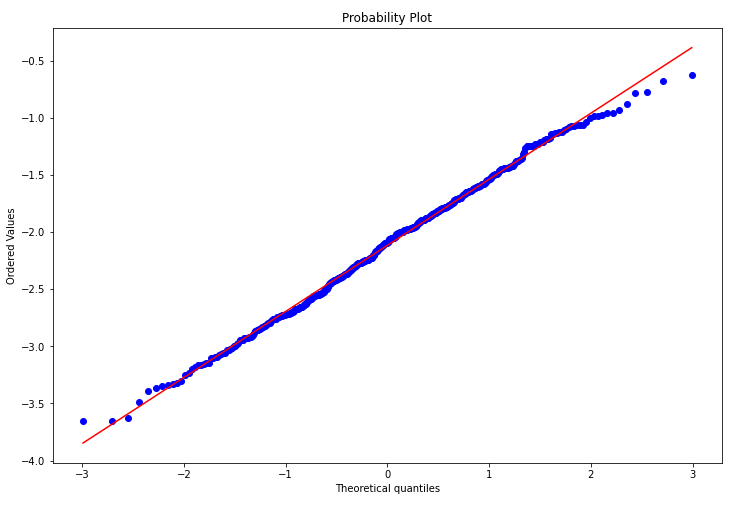图7. 转换后的帕累托数据的Q-Q图接下来再来看一个指数分布的例子。我们随机生成一个指数分布的数据集，再将其通过BOX-COX变换，转换成正态分布的数据，代码如下。state = np.random.RandomState(20) #设置随机状态
data2 = state.exponential(size = 500) #生成指数分布数据集
converted_data2 = stats.boxcox(data2) #将数据进行BOX-COX变换
然后再分别绘制原数据和经过BOX-COX变换后的数据的直方图。fig, ax = plt.subplots(figsize=[12,8])
sns.distplot(data2) #绘制原始指数分布数据的直方图
plt.show()

fig, ax = plt.subplots(figsize=[12,8])
sns.distplot(converted_data2) #绘制转换后数据的直方图
plt.show()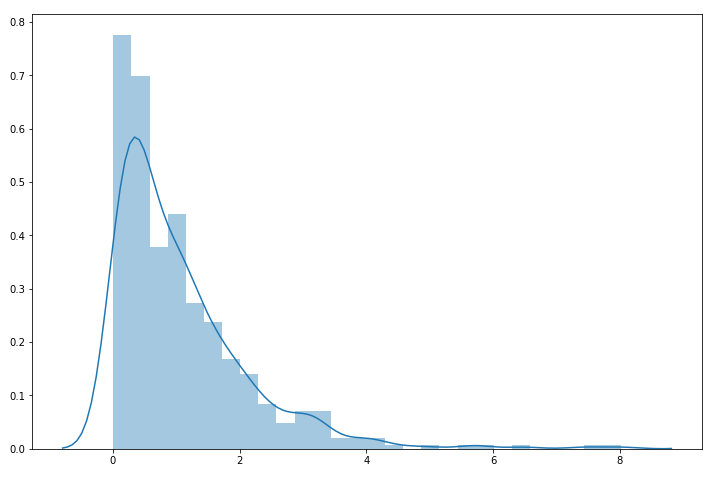图8. 指数分布数据直方图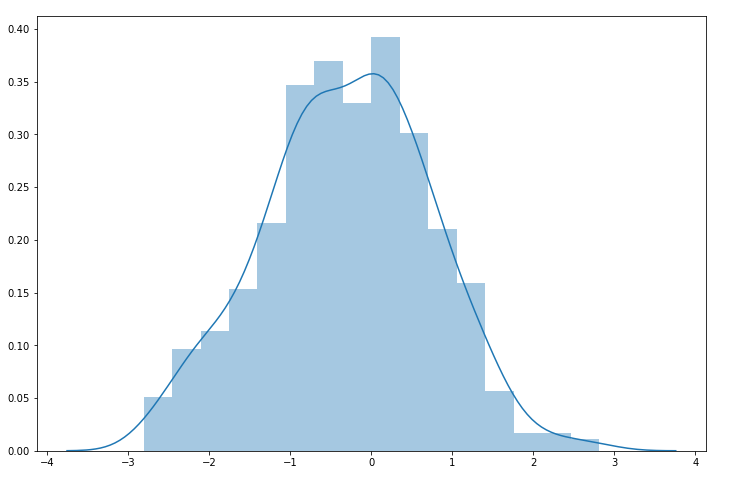图9. 转换后的指数分布数据直方图结果如图8和图9所示。通过对比图8和图9，我们可以看到数据经过转换之后，正态性明显有了改善，但我们还要验证一下。这里我们不再用前面例子中的验证方法，而是使用另外一种方法，代码如下。k1, p1 = stats.normaltest(data2)
k2, p2 = stats.normaltest(converted_data2)
这里stats.normaltest就是检验数据正态性的方法，该方法也会返回两个值，其中第二个是假设检验中常用到的P值，我们用这个值来判断数据是否具有正态性。我们得到的p1值为5.580577119276143e-57，这个值几乎为0，说明该数据不服从正态分布，而p2值为0.5322400503708197，说明该数据大概率服从正态分布。这也说明BOX-COX变换对原数据的转换效果还是很好的。本文通过两个例子来说明BOX-COX变换对非正态分布数据的转换，也介绍了一些scipy当中数据正态性验证的方法。对于BOX-COX变换更深入的使用，感兴趣的读者也可以自行查找相关资料。作者简介：Mort，数据分析爱好者，擅长数据可视化，比较关注机器学习领域，希望能和业内朋友多学习交流。赞赏作者Python中文社区作为一个去中心化的全球技术社区，以成为全球20万Python中文开发者的精神部落为愿景，目前覆盖各大主流媒体和协作平台，与阿里、腾讯、百度、微软、亚马逊、开源中国、CSDN等业界知名公司和技术社区建立了广泛的联系，拥有来自十多个国家和地区数万名登记会员，会员来自以工信部、清华大学、北京大学、北京邮电大学、中国人民银行、中科院、中金、华为、BAT、谷歌、微软等为代表的政府机关、科研单位、金融机构以及海内外知名公司，全平台近20万开发者关注。长按扫码添加“Python小助手”后回复“正态”获取本文全部代码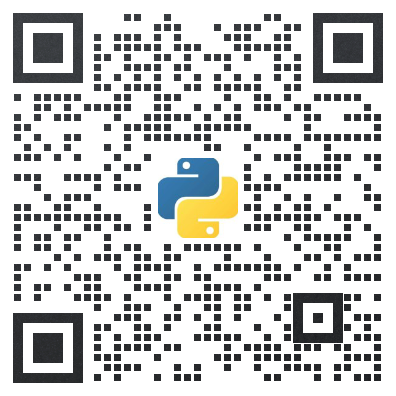▼点击成为社区会员   喜欢就点个在看吧

展开全文python 机器学习 人工智能 深度学习
• 此外，根据熵定理，自然参数一般服从正态分布（高斯分布），因此，在进行建模之前，需要对所分析的数据进行正态分布检验分析，根据数据正态分布特性判断数据采集策略及模型性能优化方法。 目前数据正态分布检验方法...
在对特定分析对象进行建模分析时，一般假设该模型变量服从正态分布，然后采用符合该变量的分析方法建立相关的分析模型。此外，根据熵定理，自然参数一般服从正态分布（高斯分布），因此，在进行建模之前，需要对所分析的数据进行正态分布检验分析，根据数据正态分布特性判断数据采集策略及模型性能优化方法。

目前数据正态分布检验方法主要分为3类：

1. 图形分析方法（graphical methods）

该类方法主要通过对分析数据建立直观图形判断是否服从正态分布，常用的图形分析方法主要有：直方图、Q-Q图及箱形图；

2. 数值方法（numerical methods）

该类方法通过检测分析数据的偏度（skewness）和峰度（kurtosis）实现正态测试；

3. formal normality test（应该翻译为正式正态检测）

公式中各个变量含义及泛函表示请参考文献《Power comparisons of Shapiro-Wilk, Kolmogorov-Smirnov,Lilliefors and Anderson-Darling tests》。

展开全文机器学习 matlab
• ## 正态性检验方法汇总

万次阅读 多人点赞 2019-05-22 10:23:46
0.概述 正态分布在统计学中有着极为... 对于正态性检验，建议首先利用直方图或核密度估计得到样本数据的分布图，若分布严重偏态或尖峰，可认为其不是来自于正态分布；若根据直方图或核密估计分布不容易做出判断，...
本文主要对正态性检验方法做了汇总，重点阐述了常用的正态性检验方法的使用场景及其在 R 或 Python 中的实现。
0.概述
正态分布在统计学中有着极为重要的地位，它是$\chi^2$分布、$t$分布、$F$分布的基础，也是许多统计方法的理论基础，故检验样本是否来自正态分布具有十分重要的意义。
正态性检验的方法有很多，以下列举了一些常见的方法：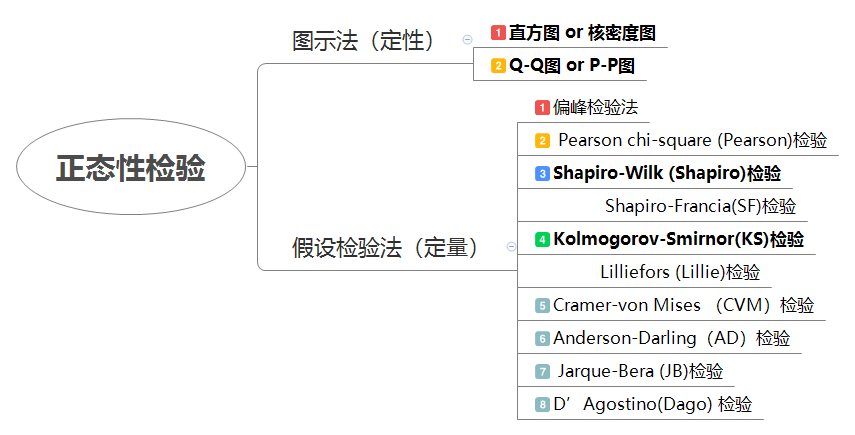对于正态性检验，建议首先利用直方图或核密度估计得到样本数据的分布图，若分布严重偏态或尖峰，可认为其不是来自于正态分布；若根据直方图或核密估计分布不容易做出判断，再利用各检验方法对其检验。
注意：对于正态性检验，应该避免仅根据一种检验方法便轻易的做出决策，应该使用多种方法，综合评价。 source:参考文献

另还有一些选择正态性检验的规定，仅供参考：

SPSS 规定：当样本含量 3≤n≤5000 时，结果以 Shapiro—Wilk(W 检验) 为准，当样本含量 n>5000 结果以 Kolmogorm —Smimov(D 检验，修正后的KS检验，即Lillie检验，见2.2.3) 为准。
SAS 规定：当样本含量 n≤2000 时，结果以 Shapim—Wilk(W 检验) 为准，当样本含量 n>2000 时，结果以 Kolmogorov—Smimov(D 检验，修正后的KS检验) 为准。

下文对直方图、Q-Q图以及Shapiro检验、KS检验的原理做一个较为详细的叙述，并给出对应方法在R语言和Python中的实现。另外，对其余方法做简要叙述。

本文内容中理论部分是作者阅读文献后整理得到

1.图示法
1.1 直方图
1.1.1 介绍
直方图(histogram)是用于展示分组数据分布的一种图形，它是用矩形的宽度和高度（即面积）来表示频数分布的。绘制该图时，在平面直角坐标系中，用横轴表示数据分组，纵轴表示频数或频率，这样就形成了一个矩形（即直方图）。
直方图与柱状图的区别：①直方图的高度与宽度都有意义。柱状图是用柱子的长度表示各类别频数的多少，其宽度（表示类别）固定；而直方图是用面积表示各组频数的多少，矩形的高度表示每一组的频数或频率，宽度则表示各组的组距；②由于分组数据具有连续性，直方图的各矩形通常是连续排列，而柱状图则是分开排列；③柱状图用于展示分类型数据，直方图用于展示数值型数据。source:参考文献
直方图的具体绘制方法见参考文献 $p_{15}$
1.1.2 直方图初判
1.2 Q-Q图检验法（直线检验法）
1.2.1 Q-Q图性质
假设样本$X_1 ,X_2 ,...,X_n$来自正态总体$N(µ,σ^2 )$. 将样本观测值按照由小到大的顺序排列, 得到次序统计量$x_{(1)} \le x_{(2)} \le ··· \le x_{(n)}$和经验分布函数
$F_n(x)=\left\{ \begin{array}{rcl} 0 & & {x < x_{(1)}}\\ k/n & & {x_{(k)}\le x \le x_{(k+1)}, k=1,2,...,n}\\ 1 & & {x\ge x{(n)}}\\ \end{array} \right.$
由于当$n$充分大时，$\forall \varepsilon >0$有$P(|F_n(x)-F(x)| >\varepsilon)=0$.
又$F(x)=P(X\le x) =\phi(\frac{x-\mu}{\sigma})$
故当$n$充分大时，$F_n(x)=\phi(\frac{x-\mu}{\sigma})$, $a.s$
即$\frac{x-\mu}{\sigma}=\phi^{(-1)}(F_n(x)), a.s$
$\forall x \in R$, 令$\phi^{-1}(F_n(x)) \equiv \omega$, 则有
$x \equiv \sigma \omega + \mu, a.s$
上式意味着，当总体是$N(\mu, \sigma^2)$且样本量$n$充分大时，样本观测值$x$和逆标准正态分布值$\omega$组成的点$(\omega, x)$几乎处于一条直线上. source: 参考文献 $p_{22}$
1.2.2 作Q-Q图的步骤

将原始数据按升序排序，得到次序统计量$x_{(1)} \le x_{(2)} \le ··· \le x_{(n)};$
计算与$x_{(n)}$相应的事件${X \le x_{(n)}}$的概率(每个数据对应的百分位数)
$p_i = F_n(x_{(n)})=\frac{i-0.5}{n};$
对概率$p_i$计算相应的标准正态分位数$u_i(i=1,2,...,n);$
把点$(u_i, x_{(i)})$画在平面直角坐标系上，并考察它们是否在一条直线上；
#计算相关系数
$r = \frac{\sum (x_{(i)}-\overline{x})(u_i-\overline{u})}{\sqrt{\sum (x_{(i)}-\overline{x})^2}\sqrt{\sum (u_i-\overline{u})^2}}$
并检验其正态性（若点$(u_i, x_{(i)})$近似在一条直线上（相关系数r=…），故可认为样本来自正态总体）

1.2.3 Q-Q图实现

R

library(car)
qqnorm(x)


Python

# QQ图
import statsmodels.api as sm
sm.qqplot(y,line='s')

# PP图
from scipy import stats
stats.probplot(x, dist="norm", plot=plt)

1.3 其他图示法

核密度图判断该样本是否来自正态总体与直方图类似。
P-P图见参考文献 $p_{24}$

2.假设检验法
建立假设
$H_0:$总体$X$的分布为正态分布$N(\mu, \sigma^2)$；(样本来自总体与正态分布无显著差异，即符合正态分布)
$H_1:$总体$X$的分布不是正态分布$N(\mu, \sigma^2)$
2.1 Shapiro-Wilk检验（Shapiro）
W 检验全称 Shapiro-Wilk 检验，是一种基于相关性的算法。计算可得到一个相关系数，它越接近 1 就越表明数据和正态分布拟合得越好。
2.1.1 检验步骤

将原始数据按升序排序，得到次序统计量$x_{(1)} \le x_{(2)} \le ··· \le x_{(n)};$
构造统计量
$W=\frac{(\sum_{i=1}^{n} a_i x_i)^2}{\sum_{i=1}^{n}(x_i - \overline{x})^2};$

注：这里， $x_i$是第 i 个顺序统计量，即第 i 个最小的样本观测值。
$a_i , i=1,2…,n$是常数，它们的定义为
$(a_1, ..., a_n) = \frac{m^TV^{-1}}{(m^TV^{-1}V^{-1}m)^{1/2}}$
$m=(m_1, ..., m_n) ^T$ 是样本顺序统计量的样本均值， V是样本
顺序统计量的协方差矩阵。
(a可直接查表得到)

计算检验统计量$W$，并与判断临界值$W_\alpha$做比较。
得出结论:若 $W < W_{\alpha}$，按表上行写明的显著性水平α舍弃正态性假设($H_0$),若 $W>W_\alpha$, 接受正态性假设.

2.1.2 实现

R

shapiro.test(x)


Python

from scipy import stats

stats.shapiro(x)

2.2 Kolmogorov-Smirnor检验（KS）
KS检验基于经验分布函数，该检验用大样本近似。其检验的是标化后的数据是否服从理论的分布。
需要注意的是样本数据如果有结点（及是重复的数据），则无法计算准确的P值。
2.2.1 KS检验步骤
设样本的经验概率分布函数定义为$F_n(x)=\frac{1}{n}\sum_{i=1}^nI_{X_i \le x}$，若$X_i \le 1$ 则$I_{X_i \le x}=1$，否则0.

构造统计量（样本的累计分布函数与理论分布的分布函数的最大值）
$D_n = \sup_{x}|F_n(x)-F(x)|$
计算检验统计量$D_n$的值，拒绝域或者 p- 值由 K-S 分布计算给出。

$D_n$的计算:
1)计算原数据的均值$\overline{x}$与标准差$s$，以及各水平对应的频数；
2)对水平的大小排序，得到频数序列，计算累计频数；
3)再计算累计频率，以及对应水平用Z-score法标准化后的取值；
4)使用标准化后的取值$x$，查表得到理论分布的值；
5)$D_n=max(累计频率 - 理论分布)$
D值越小越接近正态分布

2.2.2 KS检验实现

R

ks.test(x, "pnorm",mean(x),sd(x))
#x为要检验的样本数据，其函数默认修改的精确公式、双边检验。
#需要大样本近似命令中加exact = F；
#单边我们可以用alternative = "less"或alternative= "greater"选择。


Python

from scipy import stats

stats.kstest(x, 'norm', (x.mean(), x.std()))  # 注意，Python的ks检验其P-value有时会与其他统计软件略微不同

2.2.3 Lilliefors检验(K-S检验的修正)
Lilliefors (Lillie)检验最早由Lilliefors 于1967年提出，它是对KS正态性检验的的修正，适合大样本。
适用于一般的正态性检验。
Lillie检验实现：
library(nortest)

lillie.test(x)

# Python中未找到lillie检验的封装函数.

2.3 其他检验法

偏峰检验法
详见参考文献 $p_{21}$
Pearson chi-square (Pearson)检验
R nortest包中pearson.test()可以实现
详见参考文献 $p_{20}$ 
Cramer-von Mises （CVM）检验
R中nortest 包中的cvm.test()可以实现)，需要注意的是如果（1+0.5/n）W的值不能大于2.64
详见参考文献
详见参考文献
Jarque-Bera (JB)检验（时间序列中常用）
Python实现：

import scipy.stats as ss
ss.jarque_bera(y)

https://www.php.cn/python-tutorials-391980.html
R中可用tseries包中的jarque.bera.test()实现
详见参考文献

D’Agostino(Dago) 检验
R的fBasics包中 dagoTest()可以实现
详见参考文献
Shapiro-Francia(SF)检验
R中nortest包，sf.test()实现。是Shapiro检验的修正。
详见参考文献

还可以参考：
https://blog.csdn.net/u013524655/article/details/41053045 （SPSS）
http://www.mamicode.com/info-detail-937349.html  （R）https://blog.csdn.net/cyan_soul/article/details/81236124 （Python）

参考文献：
 何凤霞,马学俊. 基于R软件的正态性检验的功效比较[J].统计与决策,2012(18):17-19.
 贾俊平. 统计学[M]. 第6版. 北京：中国人民大学出版社, 2014.12
 高慧璇. 统计计算[M]. 北京：北京大学出版社, 1995.
 李洪成. 数据的正态性检验方法及其统计软件实现 [J]. 统计与决策, 2009(12):155-156.


展开全文R Python
• -在前面的文章中讲过，很多...描述统计方法描述统计就是用描述的数字或图表来判断数据是否符合正态分布。常用的方法有Q-Q图、P-P图、直方图、茎叶图。1. Q-Q图此Q-Q非用于聊天的QQ，Q是quantile的缩写，即分位数。...
• 什么是正态分布 关于什么是正态分布，早在中学时老师就讲过了。通俗来讲，就是当我们把数据绘制成频率直方图，所构成曲线的波峰位于中间，两边...如下图，是数据统计实例中出现的正态分布性数据： 为什么要做正...
• 正态性检验，是利用利用观测数据判断总体是否服从正态分布的检验，它是统计判决中重要的一种特殊的拟合优度假设检验。 关于正态性检验的方法主要有以下三种：直方图初判，QQ图判断，K-S检验。下面咱们一一了解： ...
• 正态性检验方法总结（1）偏度系数（2）峰度系数（3） QQ图（4）正态性W 检验 (S-W 检验)（5） 经验分布的Kolmogorov-Smirnov检验方法（K-S检验）（6）各个方法的优缺点 （1）偏度系数 样本的偏度系数（记为 g1g_{1}...机器学习 统计学 算法
• ## 如何检验数据样本的正态性？

万次阅读 多人点赞 2018-09-18 00:15:36
因此，人们在使用统计分析方法时，总是乐于正态假定，但该假定是否成立，就需要进行正态性检验了。 定义： 指如果一组观测值来自正态总体.具有正态分布的特性，就称该组观测值具有正态性。 检验方法： 1、图示法...统计学
• 判断数据正态性常用的function： 1、QQ-PLOT(quantile-quantile plot): p-value观测值和p-value期望值的比较，用于判断统计模型的合理性。 参考文献：QQ-PLOT原理详解 import statsmodels.api as sm sm.qqplot(diff_...python
• 给定序列X=(x1,x2,x3,...,xn)，判断是否该数据序列X符合正态分布。 二、方法 常见已知分布的检验方法：kstest、jbtest、lillietest、chi2gof等，这里使用使用Lilliefors检验进行描述。 语法： 1：h =...
• 正态性检验（Normality test），是利用观测数据判断总体是否服从正态分布的检验称为正态性检验。 常用的正态性检验的方法有Kolmogorov-Smirnov（K-S）法和Shapiro-Wilk（S-W）法。 本文将使用iris数据集为例，对数据...r语言 统计学
• 正态分布、正态性检验与相关性分析1 正态分布2 正态性检验2.1 直方图初判2.2 QQ图2.3 K-S检验2.3.1推导过程2.3.2 直接一行代码调用3 相关性分析3.1 图示初判3.2 Pearson相关系数3.2.1 计算推导3.2.2 代码一步到位3.3...python 算法 相关性分析
• 利用观测数据判断总体是否服从正态分布的检验称为正态性检验，它是统计判决中重要的一种特殊的拟合优度假设检验。我们可以通过直方图初步判断，QQ图判断，K-S检验 首先引入所需模块 import numpy as np import ...python 机器学习 统计学 可视化
• 题目: 某地 120 名男性红细胞数（blood）的数据（见程序中“BEGIN DATA”与“END DATA”之间的数据），此数据正态分布吗？（引自马斌荣主编书著，2001. P.150） syntax 窗口编码如下: * Ma Bin-Rong: SPSS for ...variables plot list
• 为了解决数据正态性问题，数学家们总结了很多转化方法，但是没有万能神药，都需要对症下药（根据数据的实际分布情况，选择合适的转化方法）。 下面不会介绍具体的转化方法，只是帮助大家理顺正态转化的思路，明白...
• 利用观测数据判断总体是否服从正态分布的检验称为正态性检验，它是统计判决中重要的一种特殊的拟合优度假设检验。 直方图初判 / QQ图初判 / K-S检验 1.直方图初判 import numpy as np import pandas as pd import ...python 可视化
• 上篇简要介绍了正态分布检测的必要和主要分析方法，此篇主要介绍如何通过MATLAB判断分析数据正态特性。 1. 主要方法 MATLAB检测数据正态特性主要通过数值测定和图形分析，其中数值测定指通过JB等假设检验方法...机器学习 matlab 算法
• 进行参数估计和假设检验时，通常...进行总体正态性检验的方法有很多种，以下针对MATLAB统计工具箱中提供的程序，简单介绍几种方法。 1）Jarque-Bera检验 利用正态分布的偏度g1和峰度g2，构造一个包含g1，g2的分布统
• 所以今天我们来看看，如何使用Origin进行数据的描述统计以及数据正态性检验。 图文教程 描述统计 1. 打开Origin软件，输入数据(这里有四个实验组，每个实验组有10个样本) 2. 选中数据-菜...
• 如图1所示，IBM SPSS Statistics探索性分析中的图功能包含了图表的可视化以及正态性检验，简单介绍如下： 1.箱图，以最大值、最小值、中位数和两个四分位数描述数据，可检查数据的对称性与分散程度。 2.描述性，...
• 偏度与峰度的正态性分布判断 　用统计方法数据进行分析，有很多方法如T检验、方差分析、相关分析以及线性回归等。都要求数据服从正态分布或近似正态分布。上篇博客用Q-Q图验证数据集符合正态分布。这篇博客先介绍...
• 方法一：图形-旧对话框-箱图；选择“变量”和“类别轴”（分类数据）；...方法二，可以同时分析描述统计量，百分位数，直方图等：分析-描述统计-探索；因子变量列表；统计量-百分位数；绘制- ...
• 在SPSS中，正态分布的检验方法有：计算偏度系数（Skewness）和峰度系数（Kurtosis）、Kolmogorov-Smirnov检验（KS检验或D检验）、Shapiro-Wilk（SW检验或W检验）、直方图、QQ图等。下面本葱通过...
• 验证正态分布为什么要检验数据正态性？很多时候，我们都需要使用从单一样本中获取的样本信息利用统计推断的方法来估计总体的参数信息，这是一种非常有用的统计方法，但在执行相关推断之前，我们需要验证一些假定，...
• 2正态性检验 ...因此为了保证数据满足上述统计方法的应用条件，对原始数据进行正态性检验是十分必要的。需要强调的是，一些检验理论上应该符合正态分布 （t 检验、方差分析等），但是当数据轻微的违反正态分
• 以样本的累计频率作为横坐标，以安装正态分布计算的相应累计概率作为纵坐标，把样本值表现为直角坐标系中的散点。如果资料服从整体分布，则样本点应围绕第一象限的对角线分布。 2、Q-Q图 以样本的分位数作为横......